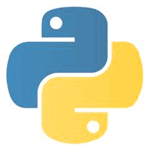# Python How to Video Pt 1If you have ever wanted to learn how to program a computer, this is the tutorial for you. I walk you step-by-step through how to do anything with Python.

Everything you can do with Python will be covered. You can watch over my shoulder as I teach programming, based off of your requests. I asked you guys what you wanted and you said:

• Go slower
• Teach me how to make an automated web site
• Let us watch you write the code

All The Code Associated with this Tutorial

﻿#!/usr/bin/python3

import math, decimal

# Demonstrate how to work with numbers in Python

def varEx():
_legalVarName = 1
Another1 = 2
yet_another = 3

print(”’and, continue, except, global, lambda, pass, while, as, def, + \
False, if, None, raise, with, assert, del, finally, import, nonlocal, + \
return, yield, break, elif, for, in, not, True, class, else, from, + \
is, or, try ”’) # \ is the line continuation character

x, y, z = 1, 2, 3

print(x, y, z)

def boolEx():
# Boolean Variables (True or False)
a = True
print(type(a))

a = 5
print(type(a))

print(bool(a))

b = True
c = False

print(b and c)

print(b or c)

print(not b)

d = (1 > 2)
print(” It is {} that 1 is greater than 2″.format(d))

def intEx():
# There are integers, binary, octal, hexidecimals

# print(d)

x = 4
y = 2

print(“x + y = “, x+y)
print(“x – y = “, x-y)
print(“x * y = “, x*y)
print(“x / y = “, x+y)
print(“x % y = “, 5%2)
print(“x // y = “, 5//2)
print(“x ** y = “, x**y)
print(“\n”)

print(“{} + {} = {}”.format(x, y, x+y))
print(“{} – {} = {}”.format(x, y, x-y))
print(“{} * {} = {}”.format(x, y, x*y))
print(“{} / {} = {}”.format(x, y, x/y))
print(“{} % {} = {}”.format(5, 2, 5%2))
print(“{} // {} = {}”.format(5, 2, 5//2))
print(“{} ** {} = {}”.format(x, y, x**y))

print(“Binary format for 4”, bin(x))
print(“divmod(5,2) = “,divmod(5,2))
print(“4 converted into a float”, float(x))
print(“Hexidecimal format for 4”, hex(x))
print(“4 raised to the power of 2”, pow(x,y))

z = 100 / 3
print(“100 divided by 3 = “, z)
print(“z is of type “, type(z))
print(“100 / 3 rounded to 3 decimals “, round(z, 3))

print(“math.ceil(100/3) = “, math.ceil(z))
print(“math.floor(100/3) = “, math.floor(z))

def floatEx(x=1, y=2):
z = x / y
print(z)
print(type(z))
print(id(z))

z = 1.1234567891011
print(z)

decimalVar = decimal.Decimal(“1.123456789101112131415”)
print(decimalVar)

decimalVar2 = decimal.Decimal(“2.345678910”)
print(decimalVar + decimalVar2)

varEx()
boolEx()
intEx()
floatEx(5, 2)

Sorry, I couldn’t get more out today. Tomorrow I’ll provide at least 2 additional video’s for the Python Video series.

If you have any questions, leave them below.

Till Next Time

Think Tank

### 2 Responses to “Python How to Video Pt 1”

1.Michael says:

Is there a zip file with the code for the Python 3 tutorial?

•Derek Banas says:

Sorry, but I made that a long time ago and didn’t think about things like that. Sorry about that